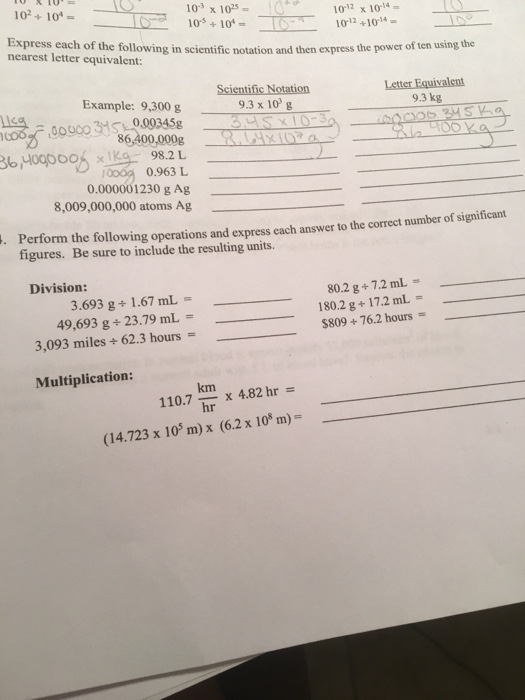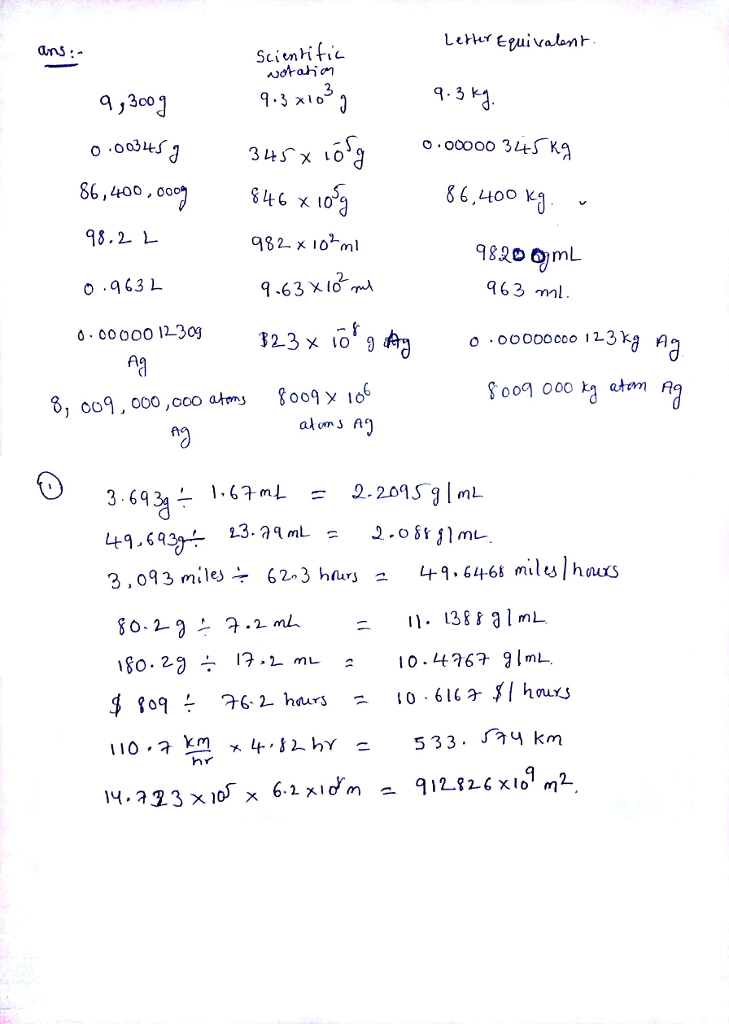# Homework Solution: Express each of the following in scientific notation and then express the power of…Express each of the following in scientific notation and then express the power of ten using the nearest letter equivalent: Perform the following operations and express each answer to the correct number of significant figures. Be sure to include the resulting units. 3.693/1.67 mL = ______ 80.2 g/7.2 mL = ____ 49,693 g/23.79 mL = ____ 180.2 g/17.2 mL = _____ 3, 093 miles/62.3 hours = ____ \$809/76.2 hours = ____ 110.7 km/hr times 4.82 hr = ____ (14.723 times 10^5) times (6.2 times 10^8 m) = _____